# Two-dimensional NMR spectroscopy

## The Nuclear Magnetic Resonance phenomena

According to the Bohr equation,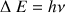, we need for inducing a transition towards the high level energy, an energy quantum with a value of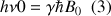.

This is a resonance condition, with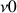which is the resonance frequency of the nucleus;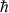the Planck constant divided by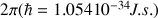and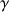the gyromagnetic ratio.

The resonance frequency of the nucleus, which is also called the Larmor frequency, is then proportional to the ﬁeld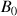value following the equation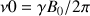.

Its range is in the high frequencies:in a ﬁeld of l.4T at the level of a frequency of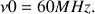This corresponds to an ultra short radio wavelength of.

1. H. Günther

La spectroscopie de RMN MASSON 1994